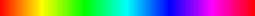### Changing Hue of Images (with Java Code)

The HSB Model

We are quite familiar with the RGB color model where all colors are formed by the mixtures of the Red, Blue and Green colors. A full measure of all the colors forms white while no color makes blue. This model however is not intuitive. Another color model is the HSB/HSV color model.

Hue:Hue

Hue is the color sensation of the light i.e. whether the color is red, blue, orange, cyan etc. This can be represented on a circle with values in degrees i.e. 0-360.

Saturation:Saturation

Saturation defines how vivid the color is i.e. how much does it differ from the corresponding shade of gray.

Brightness/Value:Brightness

Brightness as the name suggests represents the brightness of the color. A 0 brightness indicates the color is black while a brightness of 100% indicates the full bright color of corresponding hue.

We can get some really cool effects on the images by playing with HSB values. Here I elaborate on changing the hue of each pixel to a constant value.

The Pseudo Code

```for each pixel_row in image:
for each pixel in pixel_row:
R,G,B = Red, Green & Blue values of pixel
H,S,B = Convert RGB to HSB
H = The custom constant hue or shift the hue
Convert H,S,B to RGB again and save the pixel
```

The Jave Code

```// @author Abdul Fatir
// Email : abdulfatirs@gmail.com
import java.io.*;
import javax.imageio.*;
import java.awt.*;
import java.awt.image.*;
import static java.lang.System.out;
public class HueChanger
{
public static void main(String args[])throws IOException
{

int iHUE=0;
do
{
out.print("Hue (0-360):");
if(iHUE > 360 || iHUE < 0)
{
out.println("Please enter a hue value between 0-360.");
}
}
while((iHUE > 360 || iHUE < 0));
float hue = iHUE/360.0f;

BufferedImage raw,processed;
int WIDTH = raw.getWidth();
int HEIGHT = raw.getHeight();
processed = new BufferedImage(WIDTH,HEIGHT,raw.getType());

for(int Y=0; Y<HEIGHT;Y++)
{
for(int X=0;X<WIDTH;X++)
{
int RGB = raw.getRGB(X,Y);
int R = (RGB >> 16) & 0xff;
int G = (RGB >> 8) & 0xff;
int B = (RGB) & 0xff;
float HSV[]=new float;
Color.RGBtoHSB(R,G,B,HSV);
processed.setRGB(X,Y,Color.getHSBColor(hue,HSV,HSV).getRGB());
}
}
ImageIO.write(processed,"PNG",new File("processed.png"));
}
}
```